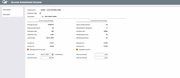# Accruing Income for an Individual Investment

 Screen ID:  Screen Title:  Panel Number:UINAC-02 Accrue Investment Income 6462Click here to magnify

Access this screen by selecting Edit on the "Work with investment accruals/work file" option from

This screen shows basic information about the selected investment, including how the monthly income calculation is set up. Notice the Accrue Thru and Accrual Amount fields at the bottom of the screen. As a batch work file was generated already, there will be figures in these fields.

If necessary, you may enter a different accrued through date here, then use Recalculate (F9) to recalculate the monthly income. You may also modify the accretion amounts for discount or premium, as appropriate.

When you are satisfied with the monthly amounts, use Save/Update  (F5) to save the changes to the work file. When you are ready, return to the previous Tool to complete additional adjustments and post the work file.

## Field Descriptions

 Field Name Description Investment ID Displays the investment ID of the selected investment. Investment Type Displays the investment type of the selected investment. Corporation This field displays the Corp ID. Income Accrual Information Principal Amount These fields show the current balance for each of the subsidiary items for this investment. Calculation Type The following codes indicate how income accruals should be calculated for this investment: Based on 365 days (365): Income will be calculated using the following formula: Coupon Rate ¸ 365 ´ Principal Value ´ No. of days since Last Accrued Date Based on 360 days, daily accrual (36D): Income will be calculated using the following formula: Coupon Rate ¸ 360 ´ Principal Value ´ No. of days since Last Accrued Date Based on 360 days, monthly accrual (36M): Income will be calculated using the following formula: Coupon Rate ¸ 360 ´ Principal Value ´ 30 days IMPORTANT: Investments with this calculation type will always accrue a full 30 days of income, even if the accrual is run more than once during the same month. Extra care should be taken when running accruals for investments with this calc. type. Based on 366 days (366): Income will be calculated using the following formula: Coupon Rate ¸ 366 ´  Principal Value ´ No. of days since Last Accrued Date Based on a fixed rate (FIX) - The accrual amount will be the same each time it is processed, using the amount shown in the Amount field described below. No accrual (NO) - No income will be calculated for this investment. Investment Rate The rate of the investment accrual. Last Accrued The date that the investment was last accrued. Accrual G/L The GL to which the accrual amounts are posted. Income G/L The GL to which the income amounts are posted. Calc Income Accrual This is used to indicate whether or not you wish to calculate income accrual for this item. This must be set to Yes in order for F9-Recalculate to work. Use No if you want to process the discount/premium accretion but not the monthly income. Accrue Thru Enter the date through which income should be accrued. Once the work file has been posted, this date will be recorded as the Last Accrued Date on the investment record. If you have created a batch work file, but wish this individual investment to use a different accrual date, change the date here and this record will use a different accrual date than the remaining records in the batch. Accrual Amount This is the amount that will be posted to the monthly income accrual subsidiary. To calculate this figure, use F9-Recalculate. You may also enter a figure manually, but be careful not to use F9 or your figure will be replaced with the system-calculated amount. Discount/Premium Information Discount G/L The GL to which the discount amounts are posted. Premium G/L The GL to which the premium amounts are posted. Calculate Accretion This is used to indicate whether or not you wish to calculate the discount/premium accretion amount for this item. This must be set to Yes in order for F9-Recalculate to work. Use No if you want to process the income accrual but not the monthly discount/premium accretion. Discount Amount This is the income amount that will be posted to the discount subsidiary. To calculate this figure (using the standard monthly amount), use F9-Recalculate. You may also enter a figure manually (or clear the amount), but be careful not to use F9 or your figure will be replaced with the system-calculated amount. Discount accretion is calculated as follows:  Discount ¸ Life in Months = Monthly Income Premium Amount This is the expense amount that will be posted to the premium subsidiary. To calculate this figure (using the standard monthly amount), use F9-Recalculate. You may also enter a figure manually (or clear the amount), but be careful not to use F9 or your figure will be replaced with the system-calculated amount. Premium accretion is calculated as follows:  Premium ¸ Life in Months = Monthly Expense

## Buttons

 Button Description Recalculate (F9) Use this to recalculate the income accrual and the discount/premium accretion using the date entered in the Accrue Thru field. ·     HINT: Because nothing is saved until F15-Add/Update is used, you could use this as a “what if” tool to show what the income/accretion amounts would be if calculated using a different accrual date. NOTE: Do not use F9 if you wish to enter an amount manually into any of the amount fields. Save/Update (F15) Use this after all changes have been made and you are satisfied with the accrual and accretion amounts displayed on the screen. The record will be saved in the work file, and you will be returned to the previous screen to select another investment.

Link to ID: https://help.cubase.org/cubase/cubase.htm#UINAC-02.htm